# 【Flutter 绘制番外】svg 文件与绘制 (中)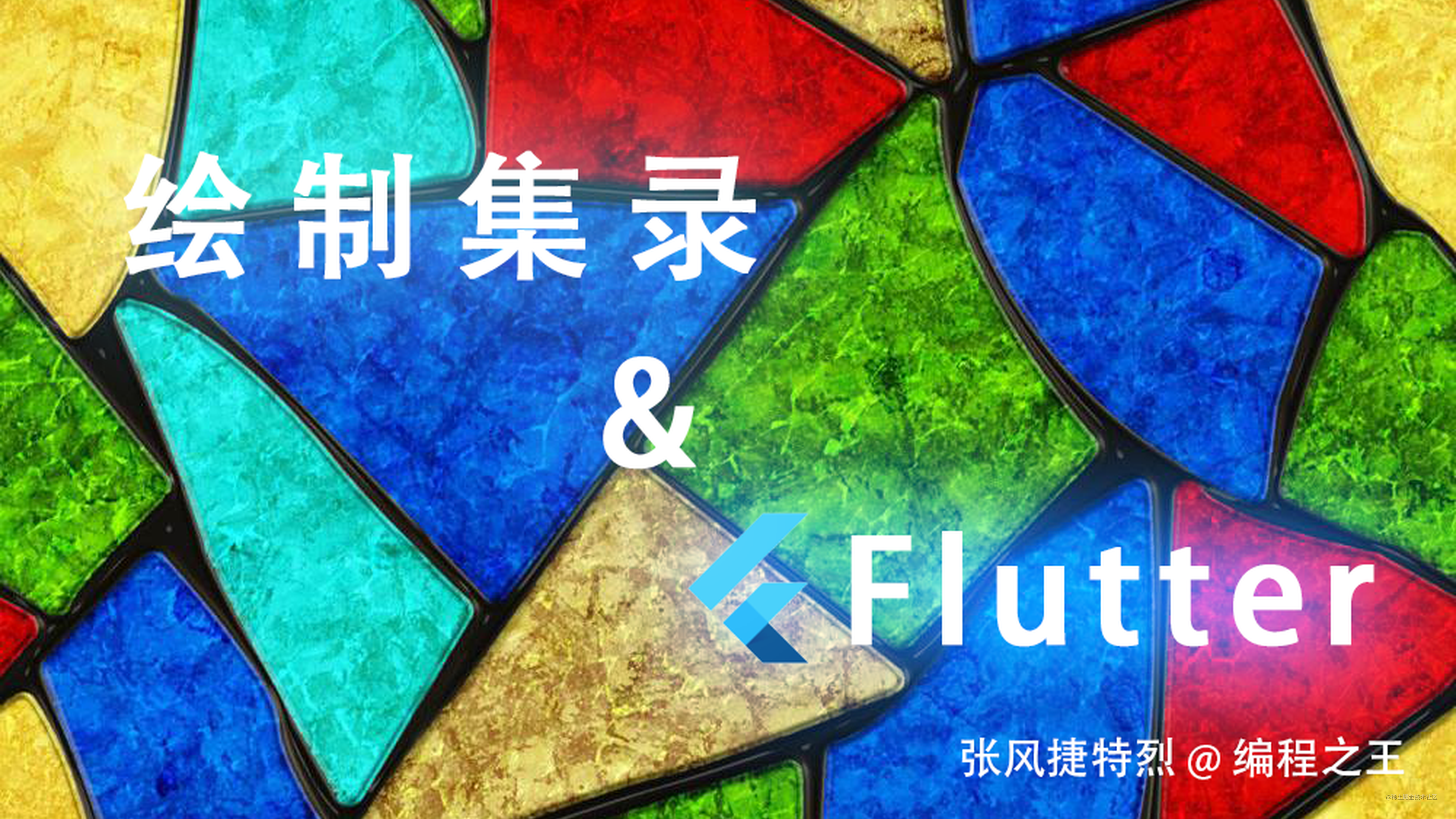##### 前言#### 一、为何要解析 svg ？``````MaskFilter.blur(BlurStyle.inner, 10)``````MaskFilter.blur(BlurStyle.solid, 20)#### 二、对 svg 解析的封装

`SVGParser` 中定义一个 `parser` 方法，解析 `src` 字符串，生成 `SVGPathResult` 列表：

``````class SVGPathResult {
final String? path;
final String? fillColor;
final String? strokeColor;
final String? strokeWidth;

SVGPathResult({
required this.path,
this.fillColor,
this.strokeColor,
this.strokeWidth,
});
}

class SVGParser {

List<SVGPathResult?> parser(String src) {
List<SVGPathResult?> result = [];
// TODO 解析 svg 文件
return result;
}
}

##### 1. svg 文件的解析

``````---->[pubspec.yaml]----
xml: ^5.3.1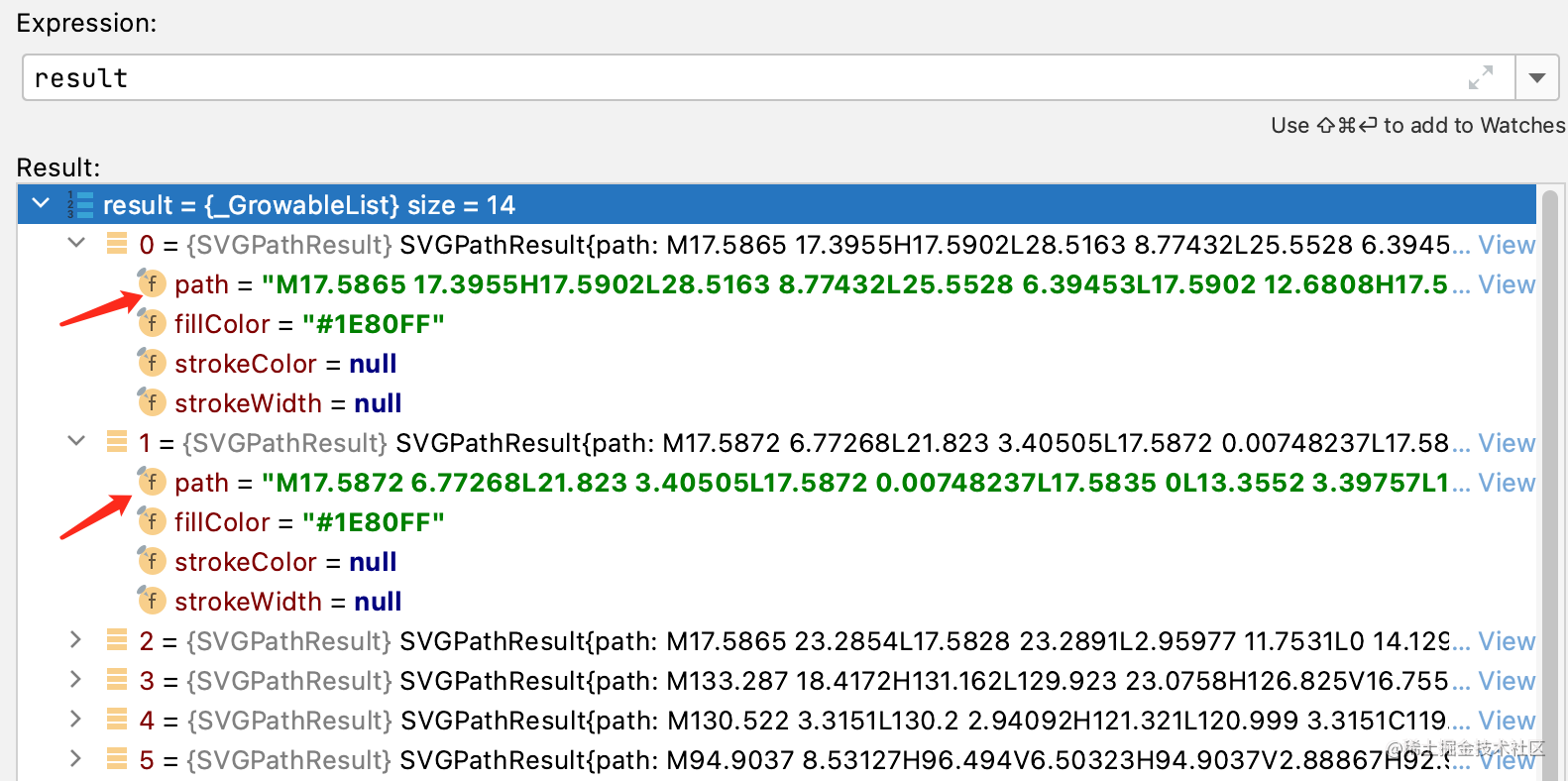``````List<SVGPathResult?> parser(String src) {
List<SVGPathResult?> result = [];
final XmlDocument document = XmlDocument.parse(src);
XmlElement? root = document.getElement('svg');
if (root == null) return result;
List<XmlElement> pathNodes = root.findAllElements('path').toList();
pathNodes.forEach((pathNode) {
String? pathStr = pathNode.getAttribute('d');
String? fillColor = pathNode.getAttribute('fill');
String? strokeColor = pathNode.getAttribute('stroke');
String? strokeWidth = pathNode.getAttribute('stroke-width');
path: pathStr,
fillColor: fillColor,
strokeColor: strokeColor,
strokeWidth: strokeWidth,
));
});
return result;
}

##### 2. svg 路径的解析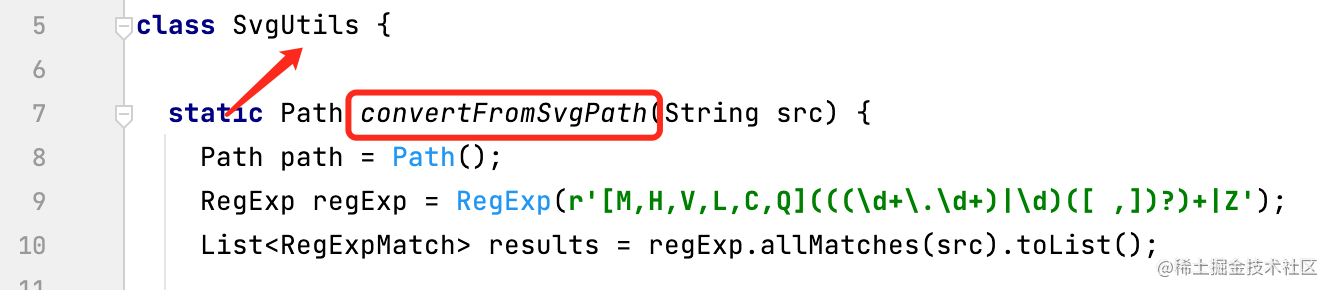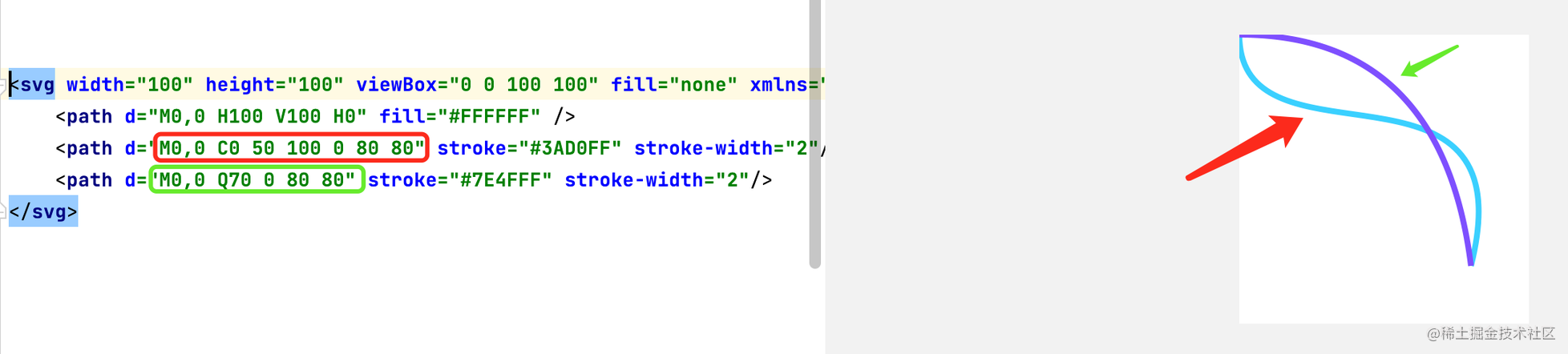``````if (op.startsWith("C")) {
List<String> pos = op.substring(1).trim().split(RegExp(r'[, ]'));
for (int i = 0; i < pos.length; i += 6) {
double p0x = num.parse(pos[i]).toDouble();
double p0y = num.parse(pos[i + 1]).toDouble();
double p1x = num.parse(pos[i + 2]).toDouble();
double p1y = num.parse(pos[i + 3]).toDouble();
double p2x = num.parse(pos[i + 4]).toDouble();
double p2y = num.parse(pos[i + 5]).toDouble();
path.cubicTo(p0x, p0y, p1x, p1y, p2x, p2y);
lastX = p2x;
lastY = p2y;
}
}

``````if (op.startsWith("Q")) {
List<String> pos = op.substring(1).trim().split(RegExp(r'[, ]'));
for (int i = 0; i < pos.length; i += 4) {
double p0x = num.parse(pos[i]).toDouble();
double p0y = num.parse(pos[i + 1]).toDouble();
double p1x = num.parse(pos[i + 2]).toDouble();
double p1y = num.parse(pos[i + 3]).toDouble();
lastX = p1x;
lastY = p1y;
}
}

##### 3.画笔的设置

`svg``path` 节点下有 `fill` 属性表示填充， `storke` 表示线条。 这些是绘制中画笔`Paint` 的属性，所有需要根据这些属性来设置画笔：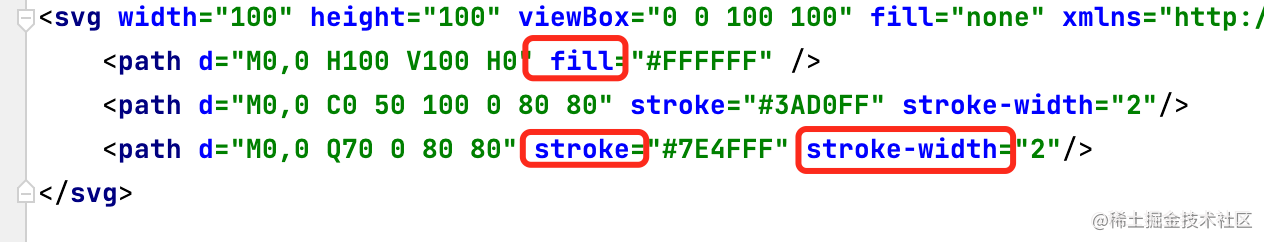``````extension SetPaintBySVGPath on SVGPathResult{
void setPaint(Paint paint){
if (this.strokeColor != null) {
paint..style = PaintingStyle.stroke;
Color resultColor = Color(
paint..color = resultColor;
}
if (this.strokeWidth != null) {
paint..strokeWidth = num.parse(this.strokeWidth!).toDouble();
}
if (this.fillColor != null) {
paint..style = PaintingStyle.fill;
Color resultColor = Color(
paint..color = resultColor;
}
}
}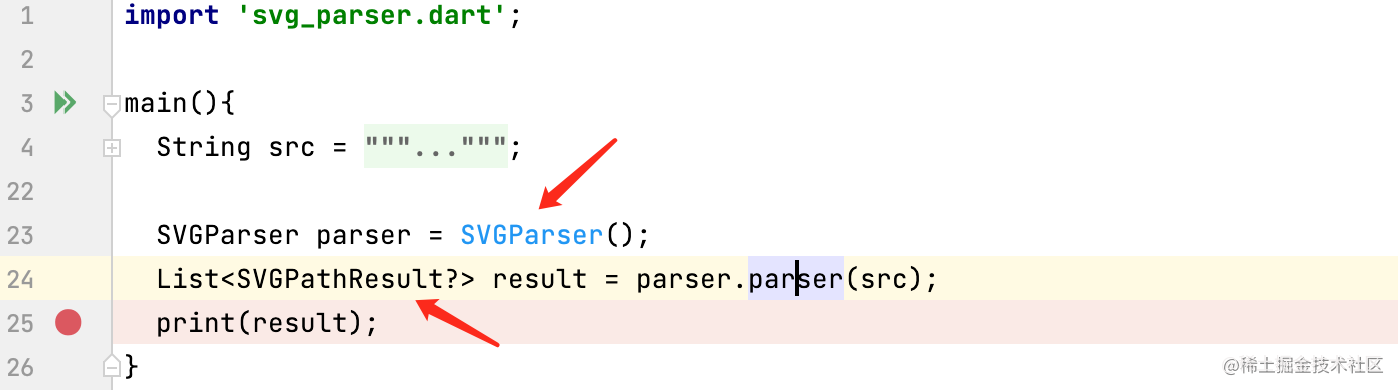#### 三、解析结果在 Flutter 中的绘制``````---->[paint]----
List<SVGPathResult?> parserResults = svgParser.parser(src);
parserResults.forEach((SVGPathResult? result) {
if (result == null) return;
if (result.path != null) {
Path path = SvgUtils.convertFromSvgPath(result.path!);
result.setPaint(mainPaint);
canvas.drawPath(path, mainPaint);
}
});``````Matrix4 matrix4 = Matrix4.diagonal3Values(0.1, 0.1, 1)
.multiplied(Matrix4.translationValues(70, 10, 0));

img,
TileMode.repeated,
TileMode.repeated,
matrix4.storage,
);``````parserResults.forEach((SVGPathResult? result) {
if (result == null) return;
if (result.path != null) {
Path path = SvgUtils.convertFromSvgPath(result.path!);
result.setPaint(mainPaint);
PathMetrics pms = path.computeMetrics();
mainPaint.style = PaintingStyle.stroke;
pms.forEach((pm) {
canvas.drawPath(pm.extractPath(0, pm.length * progress.value), mainPaint);
});
}
});

Android

Android